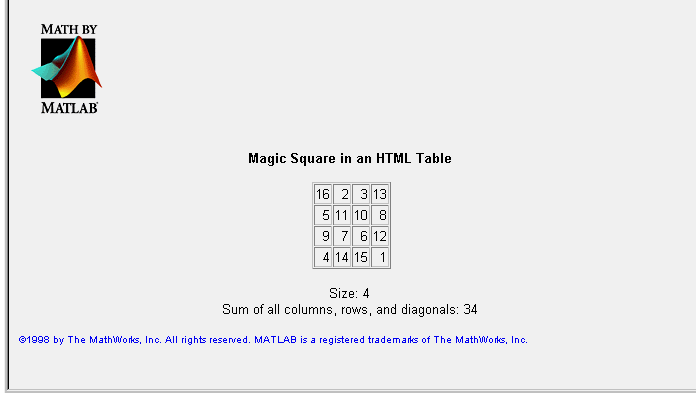MATLAB Web ServerCreating Output Documents

Output Template

The file `output_template.html` provides the code needed to create a MATLAB Web Server output document. An abbreviated version looks like

• ```<!--
Modify this file to create your own HTML output document
and save it as <MY_OUTPUT>.html, where <MY_OUTPUT> is replaced
by a name that has meaning within the context of your application.
-->
<!-- STEP 1
Display a MATLAB scalar or character string. Replace
<MY_OUTPUT_VARIABLE_1> in the following line with the name of the
MATLAB variable you want to display. Change the other text to
something meaningful within the context of your application.
-->
My output variable 1 has been computed to be
\$<my_output_variable_1>\$
<!-- STEP 2
Put all your other HTML tags here.
-->
```

webmagic Output

The `webmagic` output document contains three variables.

`\$msquare\$ `-- the completed magic square

`\$msize\$` -- the size of the magic square

`\$msum\$` -- the magic square sum along its rows, columns, or diagonals

Using `htmlrep` the `webmagic` function replaces these variables with actual values, using the input obtained from `webmagic1.html`.

The source for the `webmagic2.html` template document looks like

• ```<!--
HTML output template used by webmagic.m in call to the
function HTMLREP. (HTMLREP replaces variable names delinated
by dollar signs, e.g., \$msquare\$, with their values. It also
generates HTML tables and select lists dynamically from matrics,
cell arrays, and vectors.)
-->
<html>
<title>Magic Square in an HTML Table</title>
<div align="center">
<strong>Magic Square in an HTML Table</strong></p>
<!--
Use the MATLAB "AUTOGENERATE" HTML attribute to generate a table
dynamically from the variable "msquare", which is a matrix (in
program webmagic.m).
-->
<table border="1" cellspacing="1" autogenerate="\$msquare\$">
<tr>
<td align="right">
</td>
</tr>
</table>
<!--
Replace \$msize\$ and \$msum\$ with the contents of MATLAB variables
named "msize" and "msum" respectively as computed in webmagic.m.
-->
<p>
Size: \$msize\$<br>
Sum of all columns, rows, and diagonals: \$msum\$
</div>
```

When displayed in a browser, `webmagic2.html` looks likeCreating MATLAB Web Server M-Files Debugging Your Application